## Saturday, August 11, 2007

### Irodov Problem 1.75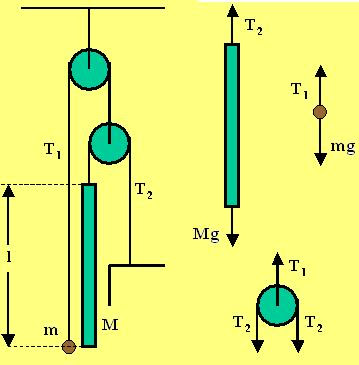Let M and m be the masses of the two rod and the bead respectively, as shown in the figure. Letbe the tension in the string holding the ball and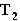be the tension in the string holding the rod. Further let,be the upward acceleration of the ball andthe downward acceleration of the rod.
Now let us consider the forces acting on the bodies.

Forces on the rod: There are two forces on the rod i) the force of gravity pulling it down Mg and the tension in the stringpulling it up. The acceleration of the rod isdownwards. Hence we have,Forces on the bead: There are two forces acting on the bead, i) the gravity mg pulling it down and ii) the tension in the stringpulling it up. The acceleration of the bead isin the upward direction. Hence we have,Forces on the moving pulley: There are three forces acting on the pulley, i) the tensions in the two parts of the stringpulling it down and ii) the tensionin the string connecting the bead pulling it up. The Pulley has no mass and accelerates at a ratedownwards (since the bead moves with an accelerationupwards). Thus, we have,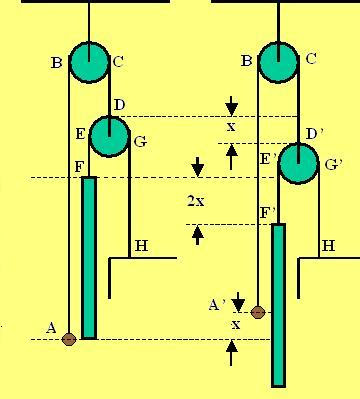Relating the accelerationsand: The acceleration of the bead and the rod are not independent of each other. The Figure shown snapshots of the system at two instants of time. AB, CD, EF and GH are the length of various sections of the strings initially and A'B, CD', E'F' and G'H are the corresponding sections of strings after some time. Suppose that the bead moves x units upwards. Then A'B = AB-x. Since the total length of the string AB + CD must be conserved, C'D' = CD +x in other wards the pulley moves x units down. Since the pulley moves x units down
the length GH reduces by x units or G'H = GH-x. Since the total length of this string GH+EF must be conserved, E'F' = EF + x. The net distance that the rod moves is the sum of distance moved by the pulley holding it and the increase in length EF. This is equal to 2x. In other words if the ball moves a distance x upwards, the rod moves 2x downwards. Thus, we have,Now we have everything need to solve the problem. From (4) and (1) we have,From (3) and (2) we have,From (5) and (6) we have,The relative acceleration of the two bodies is thus, given by,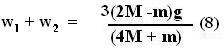The time takes to cross a distance of l (the length of the rod) is given by,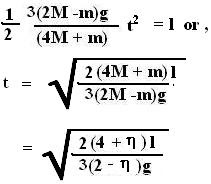BANGALORE SCHOOL OF BANKING said...

i dnt understand how a smaller mass is pulling a bigget mass up as its 1.8 times.
l b very grateful if e get any answer from u.
thanks.
sachin.
rath_sachi@yahoo.co.in

Krishna Kant Chintalapudi said...

The bead (smaller mass) is moving up and the bar is moving down. So it is the bigger mass that is pulling down the smaller mass.Akshay Ramachandran said...

I'm also preparing for IIt Jee. You have done a great job. Hats off to you. But I have a doubt. Here it is given in the question that mass of the small ball is 1.8 times the mass of rod. But still the rod is moving much faster than the rod(ITs acc is greater). Is it because of that particular consrtaint?